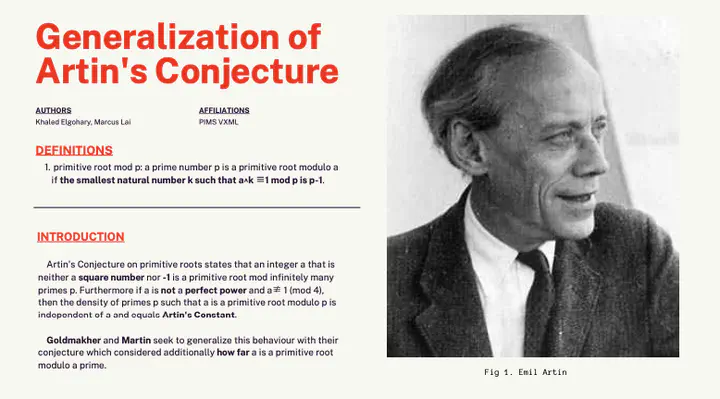# Generalizations of Artin's ConstantPoster for “Generalizations of Artin’s Constant”

• Chi Hoi Yip, University of British Columbia

• Khaled Hamdy Elgohary, University of Manitoba
• Marcus Lai, University of British Columbia

## Problem Statement

The multiplicative order of a fixed integer a modulo the prime p must divide p–1. Artin’s conjecture proposes an asymptotic formula for the number of primes for which this order equals p–1 exactly. However, one can ask for more than a yes/no answer: one can compare how close the order is to being p–1, by counting the number of prime factors of their quotient, for example. We have work in progress (with Leo Goldmakher) generalizing Artin’s conjecture to the more detailed study of this measure of closeness. In this project, students will carry out computations for lots of integers a and primes p, gathering data on the number of prime factors of (p–1 divided by the order of a modulo p). These computations can then be compared to our theoretical predictions of the relative frequencies. That comparison itself requires some work, as the predictions come in the form of a generating function that is an infinite product over primes (whose constant term is the proportion predicted in Artin’s conjecture). Students will also learn how to extract the relative frequencies from this generating function, as well as how to compute the resulting expressions to high precision.

### Final Report

This VXML project was completed by the participants listed above, as described in the following documents:

### Details

• Expected team size: 4
• Student Experience Level: Beginner: students who have taken multivariable calculus
##### Prerequisites
• Basic number theory (modular arithmetic & multiplicative order)
##### Skills
• Computational thinking skills
• Sage or similar programming languages